06-1350/Some Equations by Kurlin

(diff) ← Older revision | Latest revision (diff) | Newer revision → (diff)

Claim [Kurlin]. The function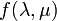$f(\lambda,\mu)$ defined by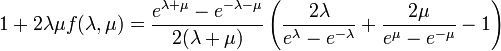$1+2\lambda\mu f(\lambda,\mu) = \frac{e^{\lambda+\mu}-e^{-\lambda-\mu}}{2(\lambda+\mu)} \left(\frac{2\lambda}{e^\lambda-e^{-\lambda}}+\frac{2\mu}{e^\mu-e^{-\mu}}-1\right)$

Satisfies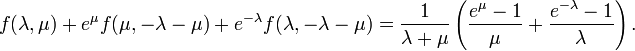$f(\lambda,\mu)+e^\mu f(\mu,-\lambda-\mu)+e^{-\lambda}f(\lambda,-\lambda-\mu) = \frac{1}{\lambda+\mu} \left(\frac{e^\mu-1}{\mu}+\frac{e^{-\lambda}-1}{\lambda}\right).$

If this class is successful, at some point in the future I will tell you why I care. But meanwhile a few questions to expose my ignorance of simple algebra. Note that$f$ is is a rational function in its variables and their exponentials. Let's call such functions "tame". The equation$f$ satisfies involves rational expressions in the variables and their exponentials and linear substitutions applied to$f$. Let us call such equations "tame". (The equation is also linear in$f$. You may or may not include this in the definition of "tame"). So we see that a tame equation has a tame solution.

• Is that always the case?
• A prety example is the tame equation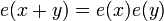$e(x+y)=e(x)e(y)$, whose solution is the "first" transcendental function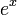$e^x$.
• Are we done defining "new" special functions after we've defined$e^x$ or are there further ones that cannot be defined in terms of it?
• If there are further ones, why haven't we heard about them? Or else, where do we read about them?
• If there aren't further ones, this is a lovely "closure" property

[Kurlin] ^  Compressed Drinfel'd Associators, V. Kurlin, arXiv:math.GT/0408398.